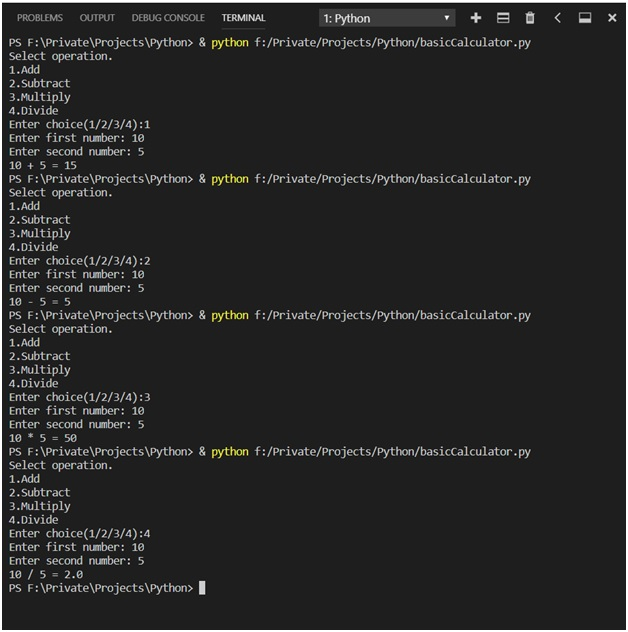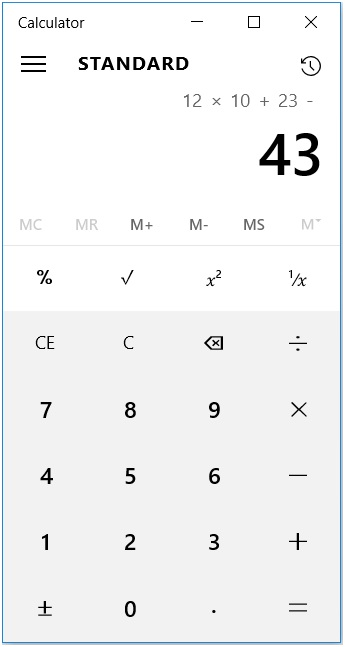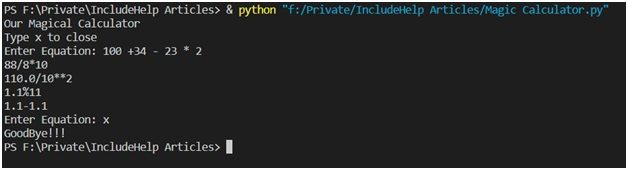# Design Traditional and Magic Calculator in Python3

Here, we are implementing Traditional (Simple) calculator and Magic Calculator using Python3.
Submitted by Himanshu Bhatt, on September 18, 2018

We will build a calculator program in this article using python3. If you search for a python calculator program on the internet, you will definitely find many of them and most of the basic calculator programs, like one below:

```# Program make a simple calculator that can
# add, subtract, multiply and divide using functions

# This function adds two numbers
return x + y

# This function subtracts two numbers
def subtract(x, y):
return x - y

# This function multiplies two numbers
def multiply(x, y):
return x * y

# This function divides two numbers
def divide(x, y):
return x / y

print("Select operation.")
print("2.Subtract")
print("3.Multiply")
print("4.Divide")

# Take input from the user
choice = input("Enter choice(1/2/3/4):")

num1 = int(input("Enter first number: "))
num2 = int(input("Enter second number: "))

if choice == '1':

elif choice == '2':
print(num1,"-",num2,"=", subtract(num1,num2))

elif choice == '3':
print(num1,"*",num2,"=", multiply(num1,num2))

elif choice == '4':
print(num1,"/",num2,"=", divide(num1,num2))
else:
print("Invalid input")
```

OutputExplanation:

This calculator program is made using simple if...else condition, we created 4 functions (addition, subtraction, multiplication, and division) for our 4 different operations. We had a print command for out basic outputs and operation variable stores the value of users choice which a number and perform an operation associated with that number and will produce the result.

Pretty interesting??? BOOO!!!!! I know this calculator is capable of doing only 4 operations and only can do one operation in its life cycle. This is what you can find on the internet, here in next section we used a better approach to build a calculator program.

## 2) Magic Calculator | A Better Approach

The thing to remember: Before we start, just know we make use Regex (regular Expression) here, so if you are not familiar with it don’t worry we got you covered, we will get through this as required of the moment.

In the modern calculator, that you have probably seen in windows and Linux these won’t ask you for choosing an operation they perform tasks you type.Image courtesy: My windows inbuilt calculator

And on hitting enter key it will produce the result, and in this section, we shall make a calculator program.

Program:

```import re

print("Our Magical Calculator")
print("Type x to close")

previous = 0
run = True
def performMath():
global run
global previous
equation = ""
if previous == 0:
equation = input("Enter Equation: ")
else :
equation = input(str(previous))

if equation == 'x':
print("GoodBye!!!")
exit(0)
else:
equation = re.sub('[a-zA-Z,:()" "]',"",equation)

if previous == 0:
previous = eval(equation)
else:
previous = eval(str(previous) + equation)

while run:
performMath()
```

OutputExplanation:

Ok... I agree it’s a lot to grasp so we will understand the above program in steps.

First, we imported the Regex library, and some output for a user and define a variable like run = True and previous = 0. In while we called our performMath() function we created before. Since while is checking run so it’s an infinite loop.

Now, performMath() is our important component of the program as it the one performing all the operations.

In the first two lines of performMath() we used global keywords so that the run and previous variable we will use in the function we referred as a global variable instead of function local variable.

If previous will be zero that is we just started our program we so our calculator will ask for input otherwise it will append in previous equation itself.

In the second if...else case, it checks if the user pressed 'x' to quit if not then we called re.sub() that the regex function which takes 3 parameters 1.pattern 2.substitute 3.string. re.sub() is substitution which replaces ‘pattern’ with ‘substitute’ in the ‘string’.

Now we will look into some basic regex expression we used above, we used [] (square brackets) because it defines the set of characters that need to include in the pattern, and we can use hyphen (-) to set the characters to range in which case we choose between a to z and A to Z including other symbols and replaced them with blank spaces just to remove them from the equation string and stored it in equation itself.

In the third if...else case, we checked if previous hold any prior calculation if not to evaluate the equation with eval() function otherwise evaluate it after appending previously calculated value with the current equation.

Note: eval() function is something we shouldn't be using (in general) in our python code, because it can evaluate anything that is passed as its parameter, meaning it can actually calculate all multiplication, division, addition and subtraction as well as exponential and the calculating remainder as well. But it has a dark side too that we need to avoid, that is eval() function can actually run any python command that passed as its parameter even if its as simple as print() it will execute the command so as the security point of view we shouldn't be using it so we used Regex here to eliminate all the letters and keep only numerical characters.

Preparation